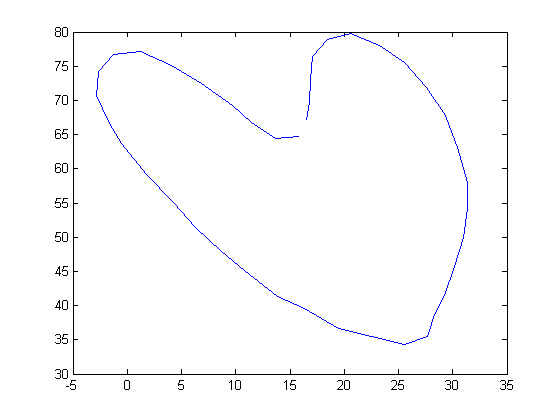## Wednesday, May 22, 2013

### Curvature: Deriving the formula based on non-length parameter.

Matching curves is an important operation in computer vision. Several methods are there. But the important thing is how we are defining the curves.

This time i am writing about some concept which uses the curvature to do this matching operation. In some of my previous posts i had written about parameterizing the curve based on length(parameter 's').It is important to understand such concepts which will help us to apply some imagination.

Curvature 'k' is the magnitude of second derivative of a curve which is parameterized using length parameter('s')
In real scenarios(live)we will not have the luxury of getting curves which follows some strict equations.Most of the cases what we get is some points(of-course again corrupted by some noise).
We need to use a numeric approximation here to find the derivatives. That is simple,the hardest part is making a list with ordered point pairs in the correct order which will closely matches the property of the original curve
Once we have such a list, it is possible to use curvature for matching. Now you may think like curvature is the magnitude of second derivative based on 's' parameter. So do we need to again parametrize the curve to 's' ? (i had this thought ). No need , we can find a curvature based on non-length parameter too.
Here it is.
Say our curve is r(s) = { x(s),y(s) }, s is from 0 to length
Now we need to find dr/dt. ( 't' is non-s parameter )
dr(s)/dt = (dr/ds) * (ds/dt) [ using chain rule) ]
= t * v (lets call (dr/ds) as tangent (t vector, not same as parameter 't' which is scalar) w.r to s, and ds/dt as 'v' which will tell how wast length is changing with respect to 't']
Now we need to find d^2r(s)/dt^2
= d(t)/dt * v + dv/dt * t
= d(dr/ds)/ds * (ds/dt) * v + (dv/dt) * t [again using chain rule of diff., first differentiate with s, because r is a function of 's' , then differentiate 's' w.r to 't']
= d(dr/ds)/ds * v * v + (dv/dt) * t
= dt/ds * v^2 + (dv/dt) * t

Here dt/ds is actually the second derivative of 'r' with respect to parameter 's'. This quantity can be written as k*n,where k is a constant and n is a unit normal vector. So the equation will become
= k*n * v^2 + (dv/dt) * t

Now take cross product between dr(s)/dt , d^2r(s)/dt^2

dr(s)/dt X d^2r(s)/dt^2 = (t*v) X (k*n*v^2 + (dv/dt) *t )
= (t*v) X (k*n*v^2) [because tXt is zero ]
= t X (k*n*v^3) [ in this only t, and n are vectors.It is very much clear.]
= (k*t*v^3) X (n)

So dr(s)/dt X d^2r(s)/dt^2 = kv^3t x n ---------------- (1)

vector t in the previous equation (1) is dr/ds, tangent vector of curve which is parameterized over 's' and is always unit length. so that means we can find 't' just by normalizing the vector 't'.

t = <dx(t)/dt,dy(t)/dt,0> / Norm[ <dx(t)/dt,dy(t)/dt,0> ]

the vectors 't' and 'n' lies in the x,y plane and also perpendicular. so take a vector 'z' which is also perpendicular to the x-y plane and its value is (0,0,1). so these three vectors (t,n,e) form a 3 dimensional coordinate system.

By using normal cross product logic we can interpret vector 'n' as cross product between e and t.

n = e x t

lets call dr(s)/dt simply as dr
lets call d^r(s)/dt^2 simply as drr

so
dr x drr = kv^3t X (e x t )
=(dr x drr)/ v^3 = k* t x (e x t )
=(dr x drr)/ v^3 = k*( e(t.t) - t(t.e))
=(dr x drr)/ v^3 = k*( e(t.t) - t(t.e))
=(dr x drr)/ v^3 = k*( e(t.t) - t(0))
=(dr x drr)/ v^3 = ke
k = (e. (dr x drr)) / v^3
k = (<0,0,1> . (dr x drr)) / v^3

Now lets find dr x drr

if we know the curve 'r' , we can easily find dr and drr. first parameterize curve to 't' , mostly all curve's natural parameterization will be t which is non-arc length parameter. Say you are drawing some a curve with mouse, then points will be placed on the order you place it right ? it may not be possible for you to place points with equal interval considering the total length of curve.
so
dr = < dx(t)/dt ,dy(t)/dt ,0 >
drr = < d^2x(t)/dt^2 , d^2y(t)/dt^2 ,0 >

dr X drr = <0,0,(dx(t)/dt) * d^2y(t)/dt^2 - dy(t)/dt * d^2x(t)/dt^2> = <0,0,dx*dyy - dy*dxx>

so k = (<0,0,1>. <0,0,dx*dyy - dy*dxx>) / v^3
k = (dx*dyy - dy*dxx>) / v^3

we need to get ride of v also. 'v' is ds/dt . what is the value of 's' , it is a function of length
s = Integrate [ Sqrt[ |dr/dt| ] ]  over the entire curve. Differentiating it again with respect to t , we get
ds/dt = Sqrt[ |dr/dt| ] = v

k = (dx*dyy - dy*dxx>) / Sqrt[ |dr/dt| ] ^3
k = (dx*dyy - dy*dxx>) / (dx^2 + dy^2)  ^(3/2)

This is the final equation for finding curvature of plane curve which is not parameterized on arc length parameter.This k is invariant to rotation, only problem lies in how efficiently we can parameterize the curve which follows the properties which we used to derive this 'k'.

see the images which shows the original curve, its curvature('k') plot, rotated image and its curvature plot. We can see that the curvature remains same.

original curve
curvature plot of original curve

Rotated curverotated curve's curvature plot

From the figures we can see that curvature remains same. we can use this feature for matching planar curves. I will post some video of that in next post.